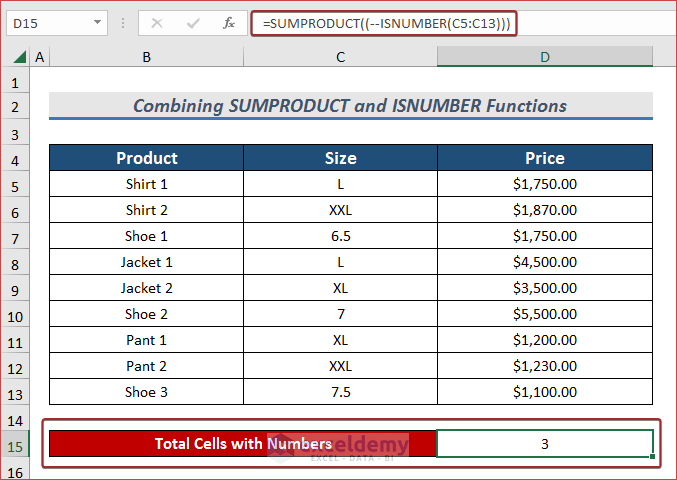# How to Count If Cell Contains Number in Excel (5 Smart Ways)

Get FREE Advanced Excel Exercises with Solutions!

If you are looking for some of the easiest ways to count if a cell contains a number in Excel then you will find this article worthy of it. Sometimes different texts, numbers, and other types of variables get mixed up in a column. In this article, I will explain step-by-step procedures to count if a cell contains a number in Excel. I hope it will be very helpful for you if you are looking for an efficient way to do so.

In order to count if a cell contains a number, we can use the COUNT, COUNTIF, SUBTOTAL, COUNTIFS, SUBTOTAL, and ISNUMBER functions.## How to Count If Cell Contains Number in Excel: 5 Smart Ways

To make it more simple to understand, I have used the following table where I have arranged some dresses in the Product, Size, and Price columns. I have both text and number variables in the Size column. Here, I would like to count the number of cells based on the numbers in this column.### 1. Use COUNT Function to Count Cells with Number

You will be able to count cells containing numbers by using the COUNT function. In this case, I will use the Size column to count the number of cells containing numbers.

Steps:

• Select a cell (i.e. D15) to have output.
• Now, insert the following formula that cell to count cells containing numbers.
`=COUNT(C5:C13)`
• Here, C5:C13 is the range of values### 2. Apply COUNTIF Function to Count Cells with Number

You will be able to count cells containing numbers by using the COUNTIF function. For more details, go through the below section.

Steps:

• Type the following formula in your preferred cell and press ENTER to count cells with numbers.
`=COUNTIF(C5:C13,"<>*")`
• Here, C5:C13 is the range of values and before the wildcard, <> is used which means Not Equal to any texts.### 3. Use SUBTOTAL function to Count Cells with Number

Another simple yet gorgeous way to count cells with numbers is to use the SUBTOTAL function. Let’s see it in detail.

Steps:

• Pick a cell for the desired output and apply the following formula for counting cells with numbers.
`=SUBTOTAL(102,C5:C13)`Read More: Excel Count Number of Cells in Range

### 4. Apply COUNTIFS Function to Count Cells with Number

In order to count cells with numbers including certain criteria, we can use the COUNTIFS function. It will satisfy all of our conditions.

Steps:

• Apply the following formula to have the total number of cells having number after fulfilling the criteria.
`=COUNTIFS(B5:B13,"*Shoe*",D5:D13,">1500")`
• Here, B5:B13 is the first criteria range and Shoe is the first criteria. Moreover, D5:D13 is the second criteria range, and “>1500” is the second criterion.### 5. Combine SUMPRODUCT and ISNUMBER Functions to Count Cells with Number

You will be able to count cells containing numbers by using the SUMPRODUCT function. But it will be assisted with the ISNUMBER function. In this case, I will use the Size column to count the number of cells containing numbers.

Steps:

• Select a cell (i.e. D15) to have output.
• Now, insert the following formula that cell to count cells containing numbers.
`=SUMPRODUCT((--ISNUMBER(C5:C13)))`
• Here, C5:C13 is the range of the ISNUMBER function.

###⧪ Formula Breakdown ⧪

–ISNUMBER(C5:C13) —> checks whether the values in those cells are text or number.
Output: {0;0;1;0;0;1;0;0;1}

SUMPRODUCT((–ISNUMBER(C5:C13)))
SUMPRODUCT(({0;0;1;0;0;1;0;0;1})) —> returns the summation of cells containing number.
Output: 3

## Conclusion

In this article, I have tried to cover 5 of the simplest ways to count if a cell contains a number in Excel effectively. Hope you will find it useful. If you have any suggestions or questions, feel free to share them with us.

## What is ExcelDemy?

ExcelDemy Learn Excel & Excel Solutions Center provides free Excel tutorials, free support , online Excel training and Excel consultancy services for Excel professionals and businesses. Feel free to contact us with your Excel problems.Tanjima Hossain

Hello everyone, This is Tanjima Hossain. I have completed my graduation from BUET. Then I have started working as a technical writer in SOFTEKO. I have grown interest in technical content writing, research topics, numerical analysis related field and so I am here. Besides this I love to interact with different people and I love to spend my spare time by reading, gardening ,cooking etc.

We will be happy to hear your thoughtsAdvanced Excel Exercises with Solutions PDF